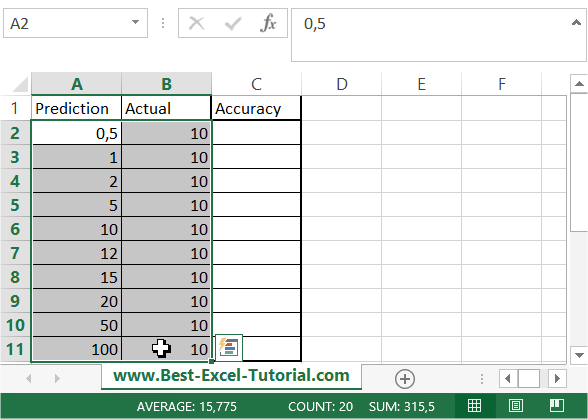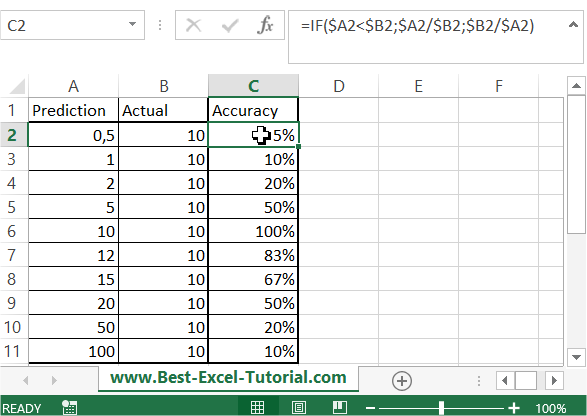#### Calculating forecast accuracy and precision

In this Excel tutorial you will teach yourself how to calculate forecast accuracy and precision.

Forecast accuracy and precision are two important metrics in forecasting, and can be calculated in Excel using a few different formulas.

Accuracy refers to how close the forecast is to actual values, and can be measured using the mean absolute deviation (MAD), mean absolute percentage error (MAPE), or mean squared error (MSE).

Precision refers to the variability or uncertainty of the forecast, and can be measured using the mean absolute deviation (MAD) or the root mean squared error (RMSE).

## Data preparation

To perform calculations you need data table.Column A is Prediction. Actual value is in column B. In C column you will calculate forecast accuracy using Excel formula.

## Forecast Accuracy Calculation:

Formula you need here is =IF(\$A2<\$B2;\$A2/\$B2;\$B2/\$A2).

Here’s a breakdown of the formula:

• IF(\$A2<\$B2, … , …) checks if the prediction in Column A is less than the actual value in Column B.
• \$A2/\$B2 calculates the accuracy when the prediction is less than the actual value.
• \$B2/\$A2 calculates the accuracy when the actual value is less than the prediction.

Copy it and paste to C2 column. Next drag and drop it down to calculate other values as well.

Final data table looks like here:#### Interpreting the Results

• A forecast accuracy value close to 100% indicates a highly accurate forecast.
• A forecast accuracy value close to 0% suggests a less accurate forecast.
• You can interpret these values to assess the quality of your forecasts.

This way you calculated forecast accuracy and precision in Excel. Thanks to it you can see how how accurate your forecasts were.

Tip: You can use simplier formula: =MIN(\$A1:\$B1)/MAX(\$A1:\$B1)

## Precision in Forecasting

Precision measures the variability or uncertainty in your forecasts. Two commonly used metrics to assess precision are Mean Absolute Deviation (MAD) and Root Mean Squared Error (RMSE). Let’s explore how to calculate these metrics in Excel.

MAD calculates the average absolute difference between your predictions and the actual values. The formula for MAD is as follows:

=SUM(ABS(\$A2:\$B2-\$B2:\$B2))/COUNT(\$A2:\$B2)

1. Select a cell in which you want to calculate MAD (e.g., cell D2).
2. Enter the formula: =SUM(ABS(\$A2:\$B2-\$B2:\$B2))/COUNT(\$A2:\$B2).

This formula computes the MAD for the first data point. To calculate MAD for the entire dataset, drag the fill handle down to apply the formula to other cells in Column D.

### Root Mean Squared Error (RMSE)

RMSE assesses precision by calculating the square root of the mean of the squared differences between predictions and actual values. The formula for RMSE is as follows:

=SQRT(SUM((\$A2:\$B2-\$B2:\$B2)^2)/COUNT(\$A2:\$B2))

Here’s how to calculate RMSE:

1. Select a cell in which you want to calculate RMSE (e.g., cell E2).
2. Enter the formula: =SQRT(SUM((\$A2:\$B2-\$B2:\$B2)^2)/COUNT(\$A2:\$B2)).

The formula calculates RMSE for the first data point. Similar to MAD, you can drag the fill handle down to compute RMSE for the entire dataset.

#### Interpreting the Results

MAD and RMSE are both measures of precision.

• A lower MAD or RMSE value indicates higher precision, meaning your forecasts are closer to the actual values with less variability.
• A higher MAD or RMSE suggests lower precision, indicating that your forecasts have more significant deviations from the actual values.